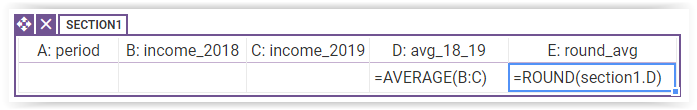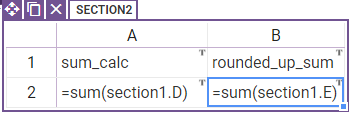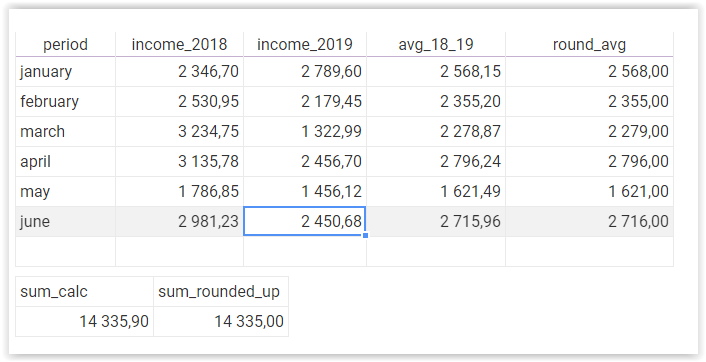# Table cell and columns addressing

In this tutorial you will learn how to address cells and columns in a table.

1.Create a new app

a)

Add a table section with 5 columns in it. Enable the 'allow add rows' option. First three columns set as editable. Set type of columns B-E to 'currency'.

b)

Give column headers names as given:period, income_2018, income_2019,avg_18_19, round_avg.

c)

Next, in 4th column use 'average' formula in columns B and C (=average(B:C)). It is a formula that calculates average value for given range of values.

d)

In the last column use 'round' formula for average value computed in column D (=round(section1.D)). This formula rounds given value up to an integer.a)

Your spreadsheet should have two columns and two rows. In first cell of column A put 'sum_calc' and in first cell of B column put 'rounded_up_sum'.

b)

In second cell of first column use 'sum' formula for column D from the previously created table (=sum(section1.D)). In second cell of second column also use this formula but for column E of the table (=sum(section1.E)). In both cells containing a formula set type 'currency'.c) test how the app works in preview modeNow, notice one important property of table columns. When we used column D in the table - in 'Round' formula we treated it as if to every new row(new cell of column E) we would copy the same 'Round' formula. Notice that this formula only rounds up one value from column D.

On the other hand when we used the same address 'section1.D' outside of the table it had a different meaning. In that case section1.D didn't mean a cell in a certain row in the table, but the whole column - all of its values. Thanks to that, we were able to sum up all values from D using one short formula =sum(section1.D).